# Table of friction coefficients

First surface Second surface Condition$\mu_s$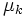$\mu_k$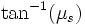$\tan^{-1}(\mu_s)$ (radians)$\tan^{-1}( \mu_s)$ (degrees)$\tan^{-1}(\mu_k)$ (radians)$\tan^{-1}(\mu_k)$ (degrees)
Aluminimum Mild steel Dry and clean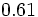$0.61$$0.47$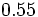$0.55$$31$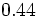$0.44$$25$
Aluminimum Aluminimum Dry and clean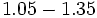$1.05 - 1.35$$1.4$$0.81 - 0.93$$46 - 53$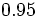$0.95$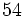$54$
Copper Steel Dry and clean$0.53$$0.36$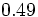$0.49$$28$$0.35$$20$
Copper Copper Dry and clean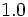$1.0$  ?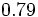$0.79$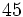$45$  ?  ?
Wood Concrete Dry and clean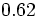$0.62$  ?$0.55$$32$  ?  ?
Wood Metal Dry and clean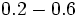$0.2 - 0.6$  ?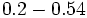$0.2 - 0.54$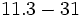$11.3 - 31$  ?  ?
Wood Metal Wet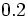$0.2$  ?$0.2$$11.3$
Wood Wood Dry and clean$0.25 - 0.5$  ?$0.24 - 0.46$$14 - 26$  ?  ?
Wood Wood Wet$0.2$  ?$0.2$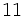$11$  ?  ?
Mild steel Mild steel Dry and clean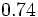$0.74$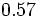$0.57$$0.64$$37$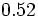$0.52$$30$
Hard steel Hard steel Dry and clean$0.78$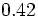$0.42$$0.66$$38$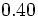$0.40$$23$

For more, see The Engineer's Handbook and Engineering Toolbox.